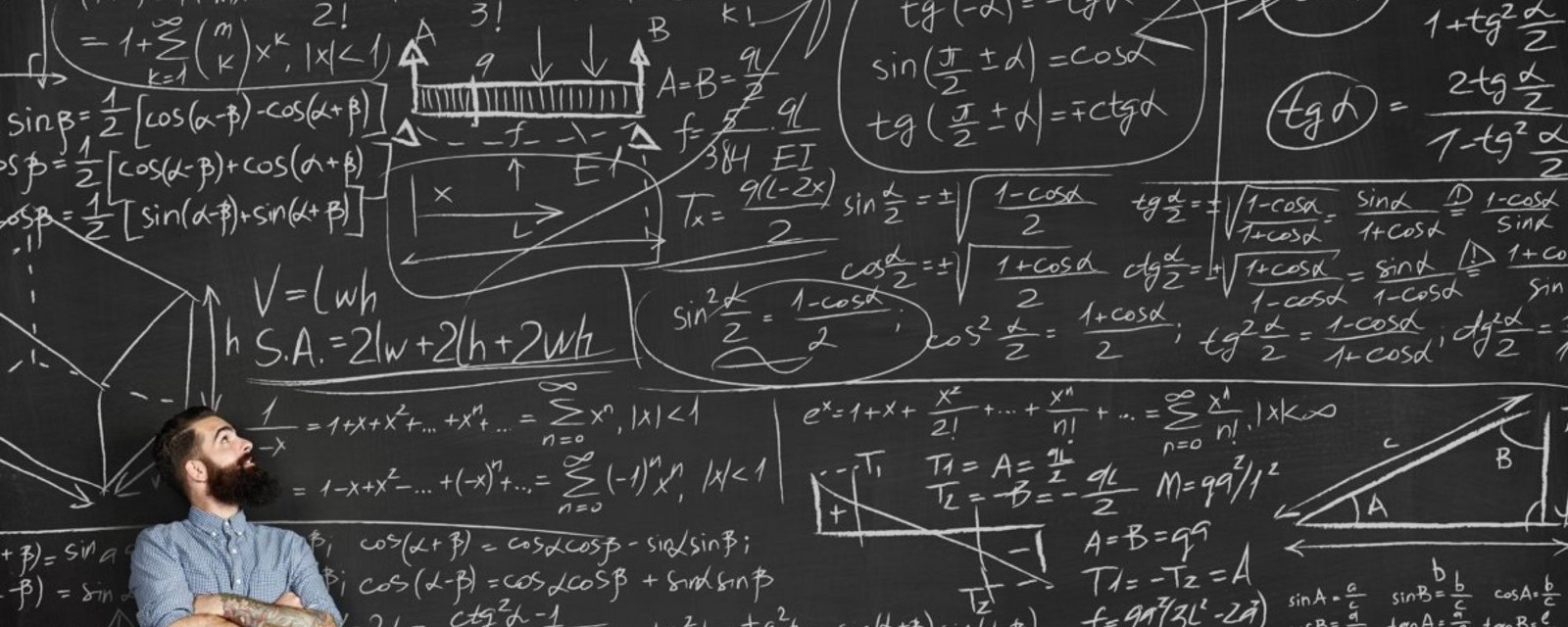# Why Is Math Important for Data Science?SHARE

Math is the base of every field of science. So it’s understandable that every technique found in data science has a deep mathematical support. We’ve written this article to show you why math is crucial for a data scientist.

We don’t have to mention the fact that you’ll need the other parts of knowledge, too, such as programming, business and the analytical and inquisitive part of the data to become the best data scientist.

If you understand math perfectly, and you understand what lays behind algorithms, you’ll use this to your advantage, and you’ll make a product recommendation for your clients. It will form some kind of edge between your peers, and it will also boost your confidence.

For newbies in the filed

People who are trying to get into this field (after spending a lot of time in other domain specifically, such as medicine, business management, retail, hardware engineering) have to deal with math. A lot of math, and not only the essential kind of math. You may think that you’ve worked enough time with sheets and numerical calculations while doing your job, but you’ll need those crucial math skills, as they’re different in data science than it was in your job.

Why science and not data?

Let’s take a clear example: web developer. This one has to deal with data every day, but it’s not actually about the modeling of that specific data. The time is pressuring, and it’s all about how you use the data, rather than explaining it. Data science should be about science, not about the data, and because of that, there are some tools that become crucial. Many of these tools are the trademarks of scientific processes. They construct hypotheses, model a process by searching the underlying dynamics and understand its limitations,  they estimate the quality of the data and quantify the ambiguity of the data. From time to time, they try to understand the mathematical evidence and the logic behind it.

This ability to think – but not in term of dry numbers, but in mathematical units and their properties – is just a part of the standard curriculum of the college – four years of them – level science degree program. You don’t have to get a diploma to have access to this type of math, but many people don’t use it or are not able to keep it in their minds at all times.

We’ve prepared a list of the topics you need to study to be the best one in data science.

Variables, functions, graphs, and equations

You get to study about series, inequalities, and sums; geometry and theorems, logarithms, rational numbers, exponential, polynomial functions, real and complex numbers, graphing and plotting, conic sections, co-ordinate systems.

You’ll work around binary search when you want to understand how a search goes faster on a million item database. To understand the dynamics in this matter, you’ll work with logarithms and equations.

Statistics

You need to know statistics if you want to get in this field. Experts from this field call the statistical learning a classical machine learning. Of course, the subject seems to be endless so it might be hard to cover the crucial concepts.

Among these, we find variance and covariance, correlation, descriptive statistics, central tendency and data summaries; probability distribution functions, in binomial, normal, uniform, central limit theorem, even chi-square, and t-distribution; the basic probability, with probability calculus, expectation, the basic idea, conditional probability and Bayes theorem. Also, random number generation, error, sampling and measurement, ANOVA and t-test, regularization and Linear regression, confidence intervals, p-values, and A/B testing.

You’ll need these in interviews. You’ll impress the employers very fast if you know the list above. If you get the job and you’ll be a data scientist, you’ll use them every day, that’s why it’s crucial to know what we’re talking about here.

SHARE